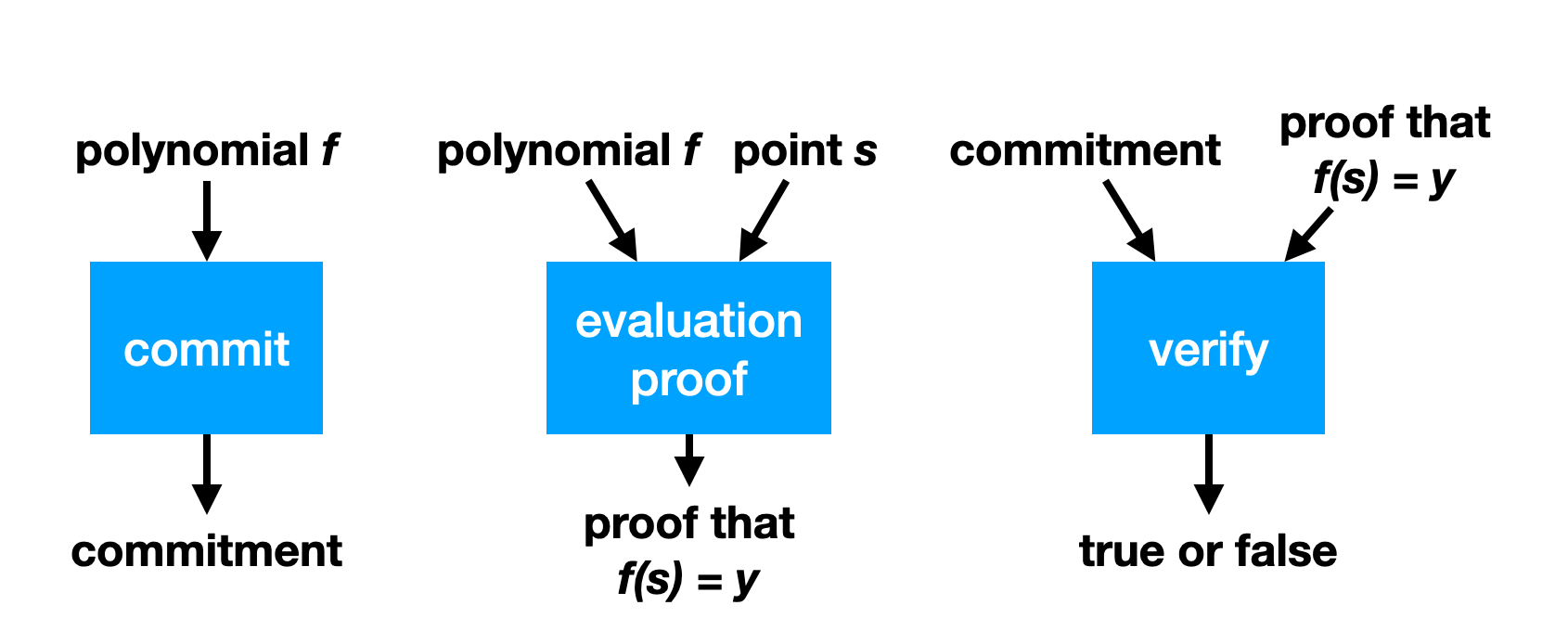# Polynomial commitments

A polynomial commitment is a scheme that allows you to commit to a polynomial (i.e. to its coefficients). Later, someone can ask you to evaluate the polynomial at some point and give them the result, which you can do as well as provide a proof of correct evaluation.## Schwartz-Zippel lemma

TODO: move this section where most relevant

Let be a non-zero polynomial of degree over a field of size , then the probability that for a randomly chosen is at most .

In a similar fashion, two distinct degree polynomials and can at most intersect in points. This means that the probability that on a random is . This is a direct corollary of the Schwartz-Zipple lemma, because it is equivalent to the probability that with